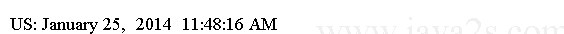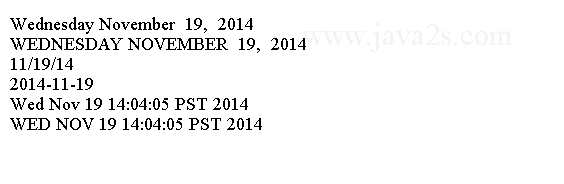# Java Format - Java printf Date/Time Format

Java printf Date/time formatting deals with date, time, and datetime values.

Java printf Date/time formatting can be applied to format values of long, Long, java.util.Calendar, java.util.Date, and java.time.temporal.TemporalAccessor types.

The value in a long type is interpreted as the milliseconds passed since January 1, 1970 midnight UTC.

The `t` uppercase variant `T` conversion characters are used to format date/time values.

The general syntax for date/time formatting is as follows:

```%<argument_index\$><flags><width><conversion>
```

Precision is not applicable to date/time formatting.

For date/time formatting, the conversion is a two-character sequence. The first character is always 't' or 'T'. The second character, which is called the conversion suffix, determines the format of the date/time argument.

The following table contains the suffix characters for Time Formatting

SuffixMeaning
HFormat time as two-digit hour of the day for the 24-hour clock. The valid values are 00 to 23. 00 is used for midnight.
IFormat a two-digit hour of the day for the 12-hour clock. The valid values are 01 to 12.
kFormat time the same as the `H` suffix except that it does not add a leading zero to the output. Valid values are 0 to 23.
lFormat time the same as 'I' suffix except that it does not add a leading zero. Valid values are 1 to 12.
MA two-digit minute within an hour. Valid values are 00 to 59.
SA two-digit second. Valid values are 00 to 60.
LA three-digit millisecond. Valid values are 000 to 999.
NA nine-digit nanosecond. The valid values are 000000000 to 999999999.
pFormat a locale-specific morning or afternoon string in lowercase. For US locale, "am" or "pm". To get "AM" and "PM", use the uppercase variant 'T' as the conversion character.
zOutput the numeric time zone offset from GMT (e.g., +0530).
ZOutput a string abbreviation of the time zone (e.g., CST, EST, IST, etc).
sOutput seconds since January 1, 1970 midnight UTC.
QOutput milliseconds since January 1, 1970 midnight UTC.

The following table list of suffix characters for Date Formatting

LetterMeaning
BOutput locale-specific full name of the month, such as "January", "February", in US locale.
bOutput locale-specific abbreviated month name, such as "Jan", "Feb", in US locale.
hSame as 'b'. Output locale-specific abbreviated month name, such as "Jan", "Feb", in US locale.
AOutput locale-specific full name for the day of the week, such as "Sunday", "Monday" for US locale.
aOutput locale-specific short name of the day of the week, such as "Sun", "Mon" for US locale.
CDivide the four-digit year by 100 and formats the result as two digits. It adds a leading zero if the resulting number is one digit. Valid values are 00 to 99. For example, if the four-digit year is 2014, it will output 20.
YOutput a four-digit year with leading zeros if the year contains less than four digits.
yOutput the last two digits of the year and adds leading zero if necessary. 2011 will output 11
jA three-digit day of the year. Valid values are 000 to 366.
mA two-digit month. Valid values are 01 to 13. The special value of 13 is required to support lunar calendar.
dA two-digit day of the month. Valid values are 01 to 31.
eDay of the month. Valid values are 1 to 31.

The following table lists of Suffix Characters for Date/Time Formatting

FormatDescription
ROutput time in 24-hour clock format as "hour:minute". It outputs the same as %tH:%tM. Examples: 11:23
TOutput time in 24-hour clock in "hour:minute:second" format. It outputs the same as "%tH:%tM:%tS". Examples 11:23:10
rOutput time in 12-hour clock format as "hour:minute:second morning/afternoon marker". It outputs the same as "%tI:%tM:%tS %Tp". Example, 09:23:45 AM
DOutput the date as "%tm/%td/%ty", such as "01/19/14"
FOutput the date as "%tY-%tm-%td", such as "2014-01-19".
cOutput the date and time as "%ta %tb %td %tT %tZ %tY", such as "Wed Jan 20 12:22:06 CST 2014".

## Example

The following code shows how to use the date time formatter. It uses '<' flag in the format specifier to reuse the value from argument.

```import java.time.LocalDateTime;
import java.time.Month;
import java.util.Locale;
//from w  w  w  . j  a  v  a 2 s. co m
public class Main {
public static void main(String[] args) {
Locale englishUS = Locale.US;
LocalDateTime ldt = LocalDateTime.of(2014, Month.JANUARY, 25, 11, 48, 16);

System.out.printf(englishUS, "US: %tB %<te,  %<tY  %<tT %<Tp%n", ldt);
}
}
```

The code above generates the following result.## Example 2

The following code formats the current date and time in the default locale. It uses a ZonedDateTime argument that holds the current date/time with time zone.

```import java.time.ZonedDateTime;
/*from  ww w .ja  v a 2  s.  c  o m*/
public class Main {
public static void main(String[] args) {
ZonedDateTime currentTime = ZonedDateTime.now();
System.out.printf("%tA %<tB  %<te,  %<tY  %n", currentTime);
System.out.printf("%TA %<TB  %<te,  %<tY  %n", currentTime);
System.out.printf("%tD %n", currentTime);
System.out.printf("%tF %n", currentTime);
System.out.printf("%tc %n", currentTime);
System.out.printf("%Tc %n", currentTime);
}
}
```

The code above generates the following result.